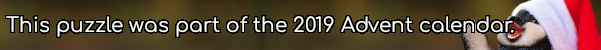mscroggs.co.uk
mscroggs.co.uksubscribe

## 10 DecemberFor all values of $$x$$, the function $$f(x)=ax+b$$ satisfies
$$8x-8-x^2\leqslant f(x)\leqslant x^2.$$
What is $$f(65)$$?
Edit: The left-hand quadratic originally said $$8-8x-x^2$$. This was a typo and has now been corrected.

## Archive

Show me a random puzzle
▼ show ▼